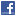# Who writes code like this?

The infamous International Obfuscated C Code winner from 1984. I have a sizeable bet that it’s Bjarne Stroustroup, the inventor of C++. However, Brian Kernighan is also a possibility. Send me your thoughts based on coding style etc.
```int i;main(){for(;i["] An explanation of the code goes something like this. int i;main(){for(;i["]i;++i){--i;}"];read('-'-'-',i+++ "hell\ o, world!\n",'/'/'/'));}read(j,i,p){write(j/p+p,i---j ,i/i);} ==== add some whitespace ========== int i; main() { for (; i["]i;++i){--i;}"]; read('-' - '-', i++ + "hello, world!\n", '/' / '/')); } read(j, i, p) { write(j / p + p, i-- - j, i / i); } ===== and char subtraced from itself is 0, and char or pointer divided by itself is 1 ===== int i; main() { for (; i["]i;++i){--i;}"]; read(0, i++ + "hello, world!\n", 1)); } read(j, i, p) { write(j / p + p, i-- - j, 1); } ======= j is always 0, p is always 1, lets remove them ====== int i; main() { for (; i["]i;++i){--i;}"]; read(i++ + "hello, world!\n")); } read(i) { write(0 / 1 + 1, i-- - 0, 1); } ======= 0 / 1 + 1 is 1, subtracting 0 does nothing, decrementing a local variable this is never used afterward also does nothing ======= int i; main() { for (; i["]i;++i){--i;}"]; read(i++ + "hello, world!\n")); } read(i) { write(1, i, 1); } ======== replace read(i) with write(1, i, 1) ===== int i; main() { for (; i["]i;++i){--i;}"]; write(1, i++ + "hello, world!\n", 1)); } ====== i[n] can be rewritten *(i + n) or *(n + i) ====== int i; main() { for (; *("]i;++i){--i;}" + i); write(1, "hello, world!\n" + i++, 1)); } === as i gets incrimented, we dereference the next char of the string which is always non-zero till we hit the null terminator, all the matters is that the string is the same length as "hello, world!\n" ===== int i; main() { for (; *("hello, world!\n" + i); write(1, "hello, world!\n" + i++, 1)); } ===== so now we can see we incriment i, printing out the next character of hello world till we hit the null terminator ==== With thanks to slashdot and Thomas Scovell (who has it tattooed on his arm)```

```Share on Facebook ```
``` technology```
``` Posts navigation ← Previous 1 … 3 4 ```